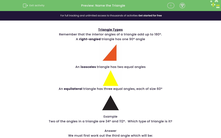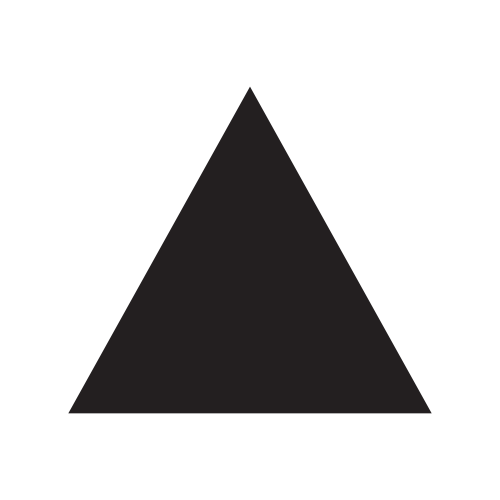# Name the Triangle

In this worksheet, students will work out the missing angle in a triangle in order to identify the type of triangle.Key stage:  KS 3

Curriculum topic:   Geometry and Measures

Curriculum subtopic:   Apply Facts About Angles and Sides

Difficulty level:#### Worksheet Overview

Remember that the interior angles of a triangle add up to 180º.

right-angled triangle has one 90º angle:An isosceles triangle has two equal angles:An equilateral triangle has three equal angles, each of size 60º:Example

Two of the angles in a triangle are 34º and 112º.  Which type of triangle is it?

We must first work out the third angle which will be:

180º - (34º + 112º) = 180º - 146º = 34º

So the triangle has angles 34º, 112º, 34º

Two angles are equal so it is isosceles.

Now, let's put these rules into practice.

### What is EdPlace?

We're your National Curriculum aligned online education content provider helping each child succeed in English, maths and science from year 1 to GCSE. With an EdPlace account you’ll be able to track and measure progress, helping each child achieve their best. We build confidence and attainment by personalising each child’s learning at a level that suits them.

Get started